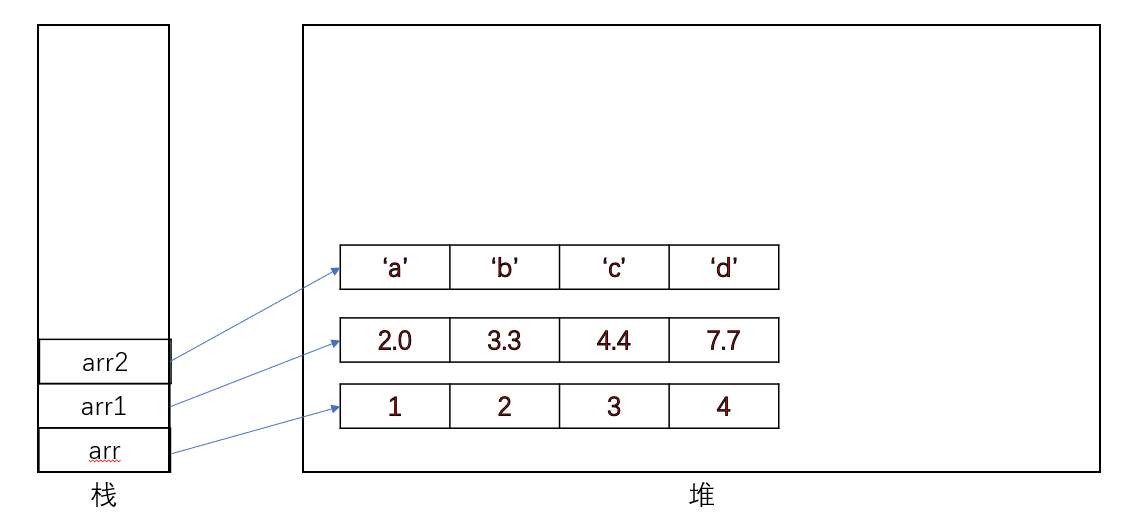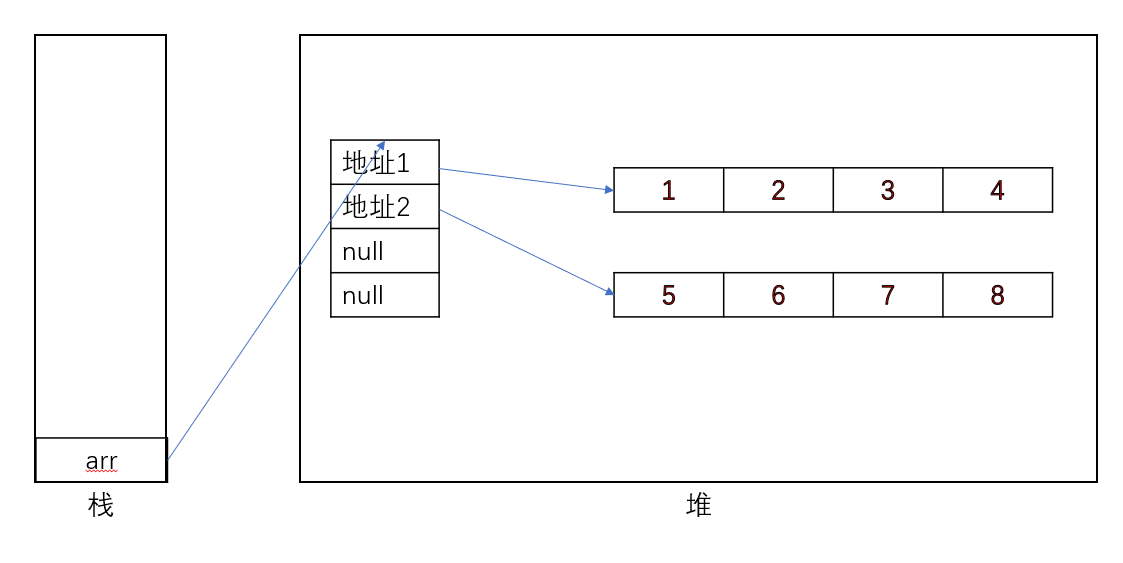## 1. 数组概述

### 1.2 数组的相关概念

• 数组名：即用来声明数组的变量名
• 元素：数组中所包含的数据的统称
• 索引（又叫角标、下标）：数组中元素的位置，从0开始依次递增
• 长度：即数组中元素的个数

### 1.3 数组的特点

1. 数组是有序排列的
2. 数组属于引用类型变量。但是数组的元素既可以是基本数据类型，也可以是引用数据类型。
3. 创建数组对象时会在内存中开辟一块连续的空间
4. 数组的长度一旦确定，则无法更改。若实际使用中需要进行长度变更，则需要重新创建一个数组。

### 1.4 数组的分类

• 按维度：一维数组、二维数组、三维数组。。。。。。
• 按数组元素类型：基本数据类型的数组、引用数据类型的数组。

## 2. 一维数组

### 2.1 一维数组的声明与初始化

• 静态初始化：初始化时直接对元素进行赋值：

int[] arr = new int[]{1, 2, 3, 4, 5};
int[] arr2 = {1, 2, 3, 4, 5};//由于new int[] 是固定的，故可以省去(Java 类型推断机制)
• 动态初始化：数组初始化与赋值操作分开进行

int[] arr3 = new int;
arr3 = 1;

String[] arr4 = new String;
arr4 = "coding";

### 2.2 一维数组元素的引用

arr = 1;
arr = 2;
arr = 3;
arr = 4;
arr = 5;

### 2.3 一维数组的属性：length

System.out.println(arr.length);

### 2.4 一维数组的遍历

for (int i = 0; i < arr.length; i++) {
System.out.println(arr[i]);
}

### 2.5 一维数组元素的默认值

• 整型：0
• 浮点型：0.0
• 布尔型：false
• 引用类型：null

### 2.6 一维数组内存结构## 3. 二维数组

### 2.1 二维数组的声明与初始化

• 静态初始化

int[][] arr = new int[][] { { 1, 2, 3 }, { 4, 5 }, { 6, 7, 8 } };
int[][] arr1 = { { 1, 2, 3 }, { 4, 5 }, { 6, 7, 8 } };

• 动态初始化

// 方式1
int[][] arr2 = new int;
// 方式2
int[][] arr3 = new int[];// 列的元素个数可以省略

### 2.2 二维数组元素的引用

System.out.println(arr1);// 先行后列
System.out.println(arr2);

### 2.3 二维数组的属性：length

System.out.println(arr.length);// 行数
System.out.println(arr[i].length);// 第 i 行元素个数，即二维数组中第i个数组的长度

### 2.4 二维数组的遍历

for (int i = 0; i < arr3.length; i++) {
for (int j = 0; j < arr3[i].length; j++) {
System.out.print(arr3[i][j] + "\t");
}
System.out.println();
}

### 2.5 二维数组元素的默认值

int[][] arr = new int;中，外层元素为arrarr等，内层元素为arrarr等。则：

• 初始化方式一int[] arr = new int

• 外层元素：地址值
• 内层元素：与一维数组初始化情况相同
• 初始化方式二int[] arr = new int[]

• 外层元素：null
• 内层元素：不可调用，会报空指针异常

### 2.6 二维数组内存结构